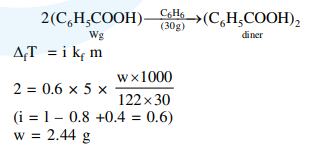Deepak Scored 45->99%ile with Bounce Back Crack Course. You can do it too!

# Solve this following

Question:

Molecules of benzoic acid $\left(\mathrm{C}_{6} \mathrm{H}_{5} \mathrm{COOH}\right)$ dimerise in benzene. 'w' $g$ of the acid dissolved in $30 \mathrm{~g}$ of benzene shows a depression in freezing point equal to $2 \mathrm{~K}$. If the percentage association of the acid to form dimer in the solution is 80 , then $\mathrm{w}$ is :

(Given that $\mathrm{K}_{\mathrm{f}}=5 \mathrm{~K} \mathrm{~kg} \mathrm{~mol}^{-1}$, Molar mass of benzoic acid $=122 \mathrm{~g} \mathrm{~mol}^{-1}$ )

1. $1.8 \mathrm{~g}$

2. $2.4 \mathrm{~g}$

3. $1.0 \mathrm{~g}$

4. $1.5 \mathrm{~g}$

Correct Option: , 2

Solution: